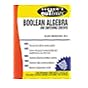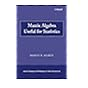Normal view MARC view ISBD view

# Matrix algebra useful for statistics / Shayle R. Searle.

Material type:TextPublisher: Hoboken, N.J. : Wiley-Interscience, c2006Description: xxii, 438 p. ; 24 cm.ISBN: 9780470009611 (pbk.); 0470009616 (pbk.).DDC classification: 512.9434
Contents:
Table of contents 1. Introduction. 2. Basic Operations. 3. Special Matrices. 4. Determinants. 5. Inverse Matrices. 6. Rank. 7. Canonical Forms. 8. Generalized Inverses. 9. Solving Linear Equations. 10. Partitioned Matrices. 11. Eigenvalues and Eigenvectors. 11A. Appendix to Chapter 11. 12. Miscellanea. 13. Applications in Statistics. 14. The Matrix Algebra of Regression Analysis. 15. An Introduction to Linear Statistical Models. References. Index.
Summary: Summary: WILEY-INTERSCIENCE PAPERBACK SERIES The Wiley-Interscience Paperback Series consists of selected books that have been made more accessible to consumers in an effort to increase global appeal and general circulation.
Tags from this library: No tags from this library for this title.Average rating: 0.0 (0 votes)
Item type Current location Collection Call number Copy number Status Date due Barcode Item holdsE-Book
E-book
Non-fiction 512.9434 SEM 2006 (Browse shelf) Not For LoanText
Reserve Section
Non-fiction 512.9434 SEM 2006 (Browse shelf) C-1 Not For Loan 25695Text
Reserve Section
Non-fiction 512.9434 SEM 2006 (Browse shelf) C-2 Not For Loan 26050
Total holds: 0
##### Browsing EWU Library Shelves , Shelving location: E-book Close shelf browser512.5 LIS 2001 Schaum's outline of theory and problems of linear algebra 512.89 MET 1970 Schaum's outline of theory and problems of Boolean algebra and switching circuits. 512.9434 AYS 1962 Schaum's outline of theory and problems of matrices / 512.9434 SEM 2006 Matrix algebra useful for statistics / 515.15 ANC 1999 Calculus : 515.15 SPS 1974 Schaum's outline of theory and problems of advanced calculus / 515.15 THE 1996 Calculus and analytic geometry /

Online version:
Searle, S. R. (Shayle R.), 1928-
Matrix algebra useful for statistics.
Hoboken, N.J. : Wiley-Interscience, c2006
(OCoLC)679404944

Includes bibliographical references (p. 429-431) and index.

Table of contents 1. Introduction. 2. Basic Operations. 3. Special Matrices. 4. Determinants. 5. Inverse Matrices. 6. Rank. 7. Canonical Forms. 8. Generalized Inverses. 9. Solving Linear Equations. 10. Partitioned Matrices. 11. Eigenvalues and Eigenvectors. 11A. Appendix to Chapter 11. 12. Miscellanea. 13. Applications in Statistics. 14. The Matrix Algebra of Regression Analysis. 15. An Introduction to Linear Statistical Models. References. Index.

Summary:
WILEY-INTERSCIENCE PAPERBACK SERIES The Wiley-Interscience Paperback Series consists of selected books that have been made more accessible to consumers in an effort to increase global appeal and general circulation.

Applied Statistics

There are no comments for this item.